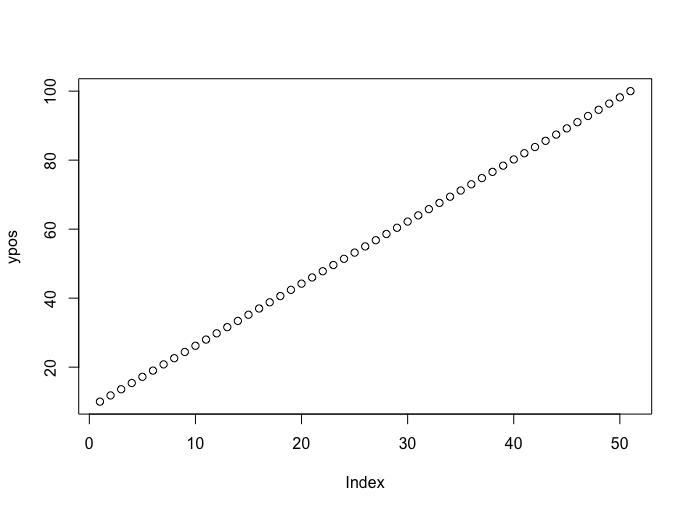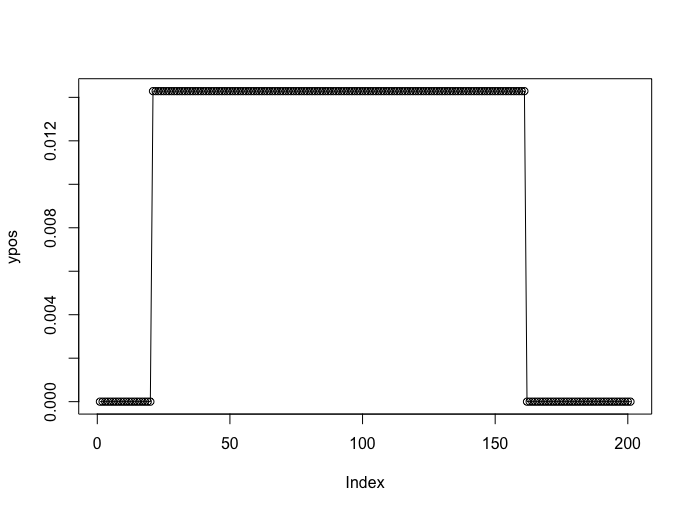# Continuous Uniform Distribution in R

• Last Updated : 06 Jun, 2021

The continuous uniform distribution is also referred to as the probability distribution of any random number selection from the continuous interval defined between intervals a and b.  A uniform distribution holds the same probability for the entire interval. Thus, its plot is a rectangle, and therefore it is often referred to as Rectangular distribution. Here we will discuss various functions and cases in which these functions should be used to get a required probability.

For uniform distribution, we first need a randomly created sequence ranging between two numbers. The runif() function in R programming language is used to generate a sequence of random following the uniform distribution.

Syntax:

runif(n, min = 0, max = 1)

Parameter:

• n= number of random samples
• min=minimum value(by default 0)
• max=maximum value(by default 1)

Example:

## R

 `print``(``"Random 15 numbers between 1 and 3"``)``runif``(15, min=1, max=3) `

Output

 “Random 15 numbers between 1 and 3”

 1.534 1.772 1.027 1.765 2.739 1.681 1.964 2.199 1.987 1.372 2.655 2.337 2.588 1.216 2.447

### Quantile for a probability

By a quantile, we mean the fraction (or percent) of points below the given value. qunif() method is used to calculate the corresponding quantile for any probability (p) for a given uniform distribution. To use this simply the function had to be called with the required parameters.

Syntax:

qunif(p,  min = 0, max = 1)

Parameter :

• p – The vector of probabilities
• min , max – The limits for calculation of quantile function

Example 1:

## R

 `min <- 0``max <- 40`` ` `print ``(``"Quantile Function Value"``)`` ` `# calculating the quantile function value``qunif``(0.2, min = min, max = max)`

Output

 “Quantile Function Value”

 8

The x values can be specified in the form of a sequence of vectors using the seq() method in R. The corresponding y positions can be calculated.

Example 2:

## R

 `min <- 0``max <- 1`` ` `# Specify x-values for qunif function``xpos <- ``seq``(min, max , by = 0.02)                      `` ` `# supplying corresponding y coordinations``ypos <- ``qunif``(xpos, min = 10, max = 100)       `` ` `# plotting the graph ``plot``(ypos) `

Output### Probability Density Function

dunif() method in R programming language is used to generate density function. It calculates the uniform density function in R language in the specified interval (a, b).

Syntax:

dunif(x,  min = 0,  max = 1,  log = FALSE)

Parameter:

• x: input sequence
• min, max= range of values
• log: indicator, of whether to display the output values as probabilities.

The result produced will be for each value of the interval. Hence, a sequence will be generated.

Example 1:

## R

 `# generating a sequence of values``x <- 5:10``print ``(``"dunif value"``)`` ` `# calculating density function``dunif``(x, min = 1, max = 20)`

Output

 “dunif value”

 0.05263158 0.05263158 0.05263158 0.05263158 0.05263158 0.05263158

All values are equal and this is the reason why it is called uniform distribution. Let us plot it for a better picture.

Example 2:

## R

 `min <- 0``max <- 100`` ` `# Specify x-values for qunif function``xpos <- ``seq``(min, max , by = 0.5)                      `` ` `# supplying corresponding y coordinations``ypos <- ``dunif``(xpos, min = 10, max = 80)       `` ` `# plotting the graph ``plot``(ypos , type=``"o"``)  `

Output### Cumulative probability distribution

The punif() method in R is used to calculate the uniform cumulative distribution function, this is, the probability of a variable X taking a value lower than x (that is, x <= X). If we need to compute a value x > X, we can calculate 1 – punif(x).

Syntax:

punif(q,   min = 0,   max = 1, lower.tail = TRUE)

All the independent probabilities that satisfy the comparison condition will be added.

Example:

## R

 `min <- 0 ``max <- 60`` ` `# calculating punif value``punif ``(15 , min =min , max = max)`

Output

 0.25

Example:

## R

 `min <- 0 ``max <- 60`` ` `# calculating punif value``punif ``(15 , min =min , max = max, lower.tail=``FALSE``)`

Output

 0.75

My Personal Notes arrow_drop_up